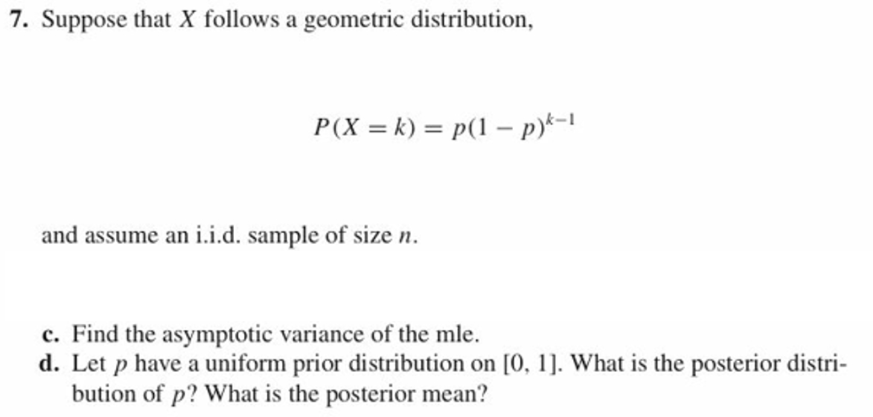7. Suppose that X follows a geometric distribution,and assume an i.i.d. sample of size nc. Find the asymptotic variance of the mle.d. Let p have a uniform prior distribution on [0, 1. What is the posterior distri-bution of p? What is the posterior mean?

Questionhelp_outlineImage Transcriptionclose7. Suppose that X follows a geometric distribution, and assume an i.i.d. sample of size n c. Find the asymptotic variance of the mle. d. Let p have a uniform prior distribution on [0, 1. What is the posterior distri- bution of p? What is the posterior mean? fullscreen
Step 1

c.

The asymptotically variance of mle:

The mle of p is given below:

Step 2

The asymptotically variance of mle is 1/I(p) is given below:

Step 3

d.

Computing posterior distribution of p and posterior mean:

The posterior distrib...

Want to see the full answer?

See Solution

Want to see this answer and more?

Our solutions are written by experts, many with advanced degrees, and available 24/7

See Solution
Tagged in

Statistics